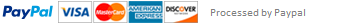### Python code completion Assignment

# 1. if-elif-else

# Fill missing pieces (____) of the following code

# such that the "print" statements make sense.

name = 'John Doe'

if ____:

print('Name "{}" is more than 20 chars long'.format(name))

length_description = 'long'

elif ____:

print('Name "{}" is more than 15 chars long'.format(name))

length_description = 'semi long'

elif ____:

print('Name "{}" is more than 10 chars long'.format(name))

length_description = 'semi long'

elif ____:

print('Name "{}" is 8, 9 or 10 chars long'.format(name))

length_description = 'semi short'

else:

print('Name "{}" is a short name'.format(name))

length_description = 'short'

# 2. for loops

# A. Fill the ____ parts in the code below.

words = ['PYTHON', 'JOHN', 'chEEse', 'hAm', 'DOE', '123']

upper_case_words = []

for ____ in words:

if ____.isupper():

____.append(____)

# B. Calculate the sum of the values in magic_dict by taking only

# into account numeric values (hint:"isinstance").

magic_dict = dict(val1=44, val2='secret value', val3=55.0, val4=1)

sum_of_values = 0

# Use a for loop here and update sum_of_values appropriately

# 3. Functions

# A. Fill inthe ____ blanks. Assume the argument is a list of integers.

____ count_even_numbers(numbers):

count = 0

for num in ____:

if ____ % 2 == ____:

count += ____

_____ _____

# B. Trasform a list of numbers to a list of descriptive strings

# using these rules:

# For odd multiples of five, the string should be 'five odd'

# For even multiple of five, the string should be 'five even'

# For odd and not a multiple of five, the string is 'odd'

# For even and not a miultiple of five, the string is 'even'

def describe_numbers(___):

# 4. Strings and lists

# A. Use str methods to convert ugly to wanted pretty.

ugly = ' tiTle of MY new Book\n\n'

# Your implementation: use str methods on ugly

pretty = 'TODO'

# B. Number formatting -- show pi to 4 decimal places: fill in the ___ blank

from math import pi

pi_to_four = '___'.format(pi)

# C. Lists amd sorting

# Take these three lists and create a single list, sorted descending

# Use list methods! Hint: help(sorted)

list1 = [6, 12, 5]

list2 = [6.2, 0, 14, 1]

list3 = [0.9]

my_sorted_list = ___

# 5. Dictionaries

# A. Create a dicitonary with keys that are the first 5 positive integers

# and the values are the squares of those numbers

square_integers = {___}

# B. Create a dict of the cubes of the next 5 integers, and then merge that

# with the first dictionsarys using the dict.update() method.

# Hint: reread the notebook for Lecture 4!

squares_and_cubes = {___}

This assignment has been answered 4 times in private sessions.